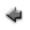previous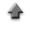home

# 3D scatter plot and fitting

You can draw 3 dimensional scatter plot. To start, click "3D Scatter Plot and Fit" tab on the application. You can also fit an arbitrary function model f(x,y,z) to a data set.
If you use the sample data, click here, and save it in the appropriate directory (or folder).

When you fit a function (in this case, a Gaussian function) to the sample data,

1. Click "Open data file", and specify the data file prepared according to the above instruction.
2. Select "unknown errors" at the line "Data type".
3. When clicking the "Plot" button bellow, the plot is displayed.
4. When clicking the "Fit" button in the window, a result of fitting is displayed.

## Data

1. Data must be inputted by normal width.
2. When no errors are included in data, the data must be inputted in order of x, y and z which the values are delimited by space or tab. Change the line, and input the data of the following row similarly until the input of the last data ends.
 x1 y1 z1 x2 y2 z2 . . . . . . . . . xn yn zn
3. When errors are included in z data only, the data must be inputted in order of x, y,z and the error of z (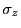) which are delimited by space or tab. Change the line, and input the data of the following row similarly until the input of the last data ends.
 x1 y1 z1 σz1 x2 y2 z2 σz2 . . . . . . . . . . . . xn yn zn σzn
4. When errors are included in all x, y and z, the data must be inputted in order of x, the error of x (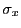), y, the error of y (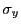), z and the error of z () which are delimited by space or tab. Change the line, and input the data of the following row similarly until the input of the last data ends.
 x1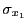y1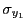z1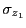x2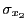y2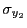z2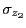. . . . . . . . . . . . . . . . . . x2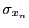y2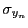zn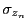5. In addition, you can use the table data form in the data file. You may include the column data not used to draw the graph in the data file. In that case, the specification of the column in the data file used to draw the graph is needed at the line "Data columns" in the following "Input" instruction.

## Inputs

1. Click "Open data file", and specify the data file prepared according to the above instruction.
2. When the labels for data are used, check the "Data labels".
3. The columns in the data file to be used to draw a plot can be specified at the line "Data columns".
• When "unknown errors" is selected at the line "Data type", the columns must be specified in order of the column of x, y, z which are delimited by space. For instance, when x are put in the third column, y in the fifth column and z in the seventh column in the data file, specify "3 5 7", and check the "Data columns" box.
• When "z errors only" is selected at the line "Data type", the columns must be specified in order of the column of x, y, z and the errors of z which are delimited by space. For instance, when x are put in the third column, y in the fifth column, z in the seventh column and the errors of z in the eighth column in the data file, specify "3 5 7 8", and check the "Data columns" box.
• When "all errors included" is selected at the line "Data type", the columns must be specified in order of the column of x, the errors of x, y, the errors of y, z and the errors of z which are delimited by space. For instance, when x are put in the third column, the errors of x in the forth column, y in the fifth column, the errors of y in the sixth column, , z in the seventh column and the errors of z in the eighth column in the data file, specify "3 4 5 6 7 8", and check the "Data columns" box.
4. The range for the x axis in the plot can be specified at the line "x range". In that case, specify the range for x axis (the numerical values must be delimited by space), and check the "x range" box. For instance, when you want to assume the range for x to be 0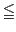x15, specify "0 15", and check the "x range" box.
5. Like the range for the x axis, the range for the y axis in the plot can be specified at the line "y range". In that case, specify the range for y in the plot (the minimum and the maximum values must be delimited by space), and check the "y range". For instance, when you want to assume the range for y in the histogram to be 0y15, specify "0 15", and check the "y range",.
6. The range for z axis in the plot can be specified at the line "z range". In that case, specify the range for z in the plot (the minimum and the maximum values must be delimited by space), and check the "z range". For instance, when you want to assume the range for z in the histogram to be 0z15, specify "0 15", and check the "z ange".
7. Select the data type of the data set at the line "Data type" according to the combinations of the errors of x, y and z.
• When no errors are included in all data, select "unknown errors".
• When the errors are included in z data only, select "z errors only".
• When errors are included in all data, select "all errors included".
8. The title for the plot can be specified at the line "Graph title". Click the "Set" button after describing it.
9. The label of the x axis in the plot can be specified at the line "Label of x axis". Click the "Set" button after describing it.
10. The label of the y axis in the plot can be specified at the line "Label of y axis". Click the "Set" button after describing it.
11. The label of the z axis in the plot can be specified at the line "Label of z axis". Click the "Set" button after describing it.
12. The explanatory note (legend) of the plot can be specified at the line "Legend of data". Click the "Set" button after describing it.

## Outputs

1. The plot can be displayed when the "Plot" button is clicked.
2. When the "Show contour" button is clicked, the contour of the plot is drawn.

## Fitting a function to data

You can fit a function model to data.
1. Input a function model to be fitted at the line "Function", and click the "Set" button. About the input of the function, refer here.
• When "unknown errors" or "z errors only" is selected at the line "Data type", you can fit a function to the plot using the method of least squares.The input of the function must follow the form z=f(x,y).
• When "all errors included" is selected at the line "Data type", you can fit a function to the plot using the method of maximum likelihood. The input of the function must follow the form f(x,y,z)=0.
2. This fitting algorithm requires the specification of initial values of the parameters included in the function model. Then, you must input the initial values of the parameters at the line "Parameters" which must be delimited by space, and click the "Set" button.
3. The range for x data to be fitted can be specified at the line "x data range". In that case, specify the range for x axis (the numerical values must be delimited by space), and check the "x data range" box. For instance, when you want to assume the range for x to be 0x15, specify "0 15", and check the "x data range" box.
4. Like the range for x data, the range for y data to be fitted can be specified at the line "y data range". In that case, specify the range for y in the plot (the minimum and the maximum values must be delimited by space), and check the "y data range". For instance, when you want to assume the range for y in the histogram to be 0y15, specify "0 15", and check the "y data range".
5. The explanatory note (legend) for the function can be specified at the line "Legend". Click the "Set" button after describing it.
6. When you use the second order derivatives of the function with respect to the parameters in computation of the parameter's errors, check the "2nd order derivatives" box.
7. When clicking the "Fit" button, the resul of fitting can be displayed, and the fitted function is also drawn on the graph.
8. When the "Show error matrix" button is clicked, the elements of the error matrix is displayed.
9. When the "Show residual plot" button is clicked, the plot of the residuals is displayed.
10. When the "Shoe residuals" button is clicked, the data of the residuals is displayed.

### Drawing a function represented by a parametric variable

You can draw a parametric function in the plot. About the function represented by parametric variables, refer here.
1. Input the parametric variable function for x at the line "parametric x", and click the "Set" button. Where the parameter names are x and y, and the constant name is p0 and p1, and so on.
2. If the constants (p0, p1, etc) are included in the parametric variable function of x, input the values delimiting by space at the "constants for x", and click the "Set" button.
3. Input the parametric variable function for y at the line "parametric y", and click the "Set" button. Where the parameter names are x and y, and the constant name is p0 and p1, and so on.
4. If the constants (p0, p1, etc) are included in the parametric variable function of y, input the values delimiting by space at the "constants for y", and click the "Set" button.
5. Input the parametric variable function for z at the line "parametric z", and click the "Set" button. Where the parameter names are x and y, and the constant name is p0 and p1, and so on.
6. If the constants (p0, p1, etc) are included in the parametric variable function of z, input the values delimiting by space at the "constants for z", and click the "Set" button.
7. Specify the range for the parameter(parametric variable) x included in the parametric variable functions of x, y and z at the "latitude range" which the values must be delimited by space, and click the "Set" button.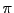(=3.14159...) can be specified as a unit other than the numerical value. For example, it can be specified as "2*pi", "1.5*pi", and so on.
8. Specify the range for the parameter(parametric variable) y included in the parametric variable functions of x, y and z at the "longitude range" which the values must be delimited by space, and click the "Set" button.
9. Click the "Draw parametric function" button.

Kazushi Neichi